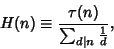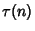Ore's Conjecture

Define the Harmonic Mean of the Divisors ofwhereis the Tau Function (the number of Divisors of). Ifis a Perfect Number,is an Integer. Ore conjectured that ifis Odd, thenis not an Integer. This implies that no Odd Perfect Numbers exist.